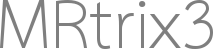# Screen normal calculation is not adapted to perspective rendering

Hey,

while adapting mrview slice mode rendering to perspective projection I discovered that the calculation of the screen normal in the projection class does the following:

``````return Eigen::Vector3f (iMVP(0,2), iMVP(1,2), iMVP(2,2)).normalized().eval();
``````

I do not fully understand the semantics of this line.
While reading about transformation matrices I read that normals are transformed by using the inverse of the transpose of a matrix instead of the matrix itself so that the scaling is handled correctly. This is explained here.

Therefore I tried changing the normal calculation to using the inversed transposed matrix. This is the same as using the 3rd row of the MVP matrix instead of the 3rd column of iMVP. I changed the line to

``````return Eigen::Vector3f (MVP(2,0), MVP(2,1), MVP(2,2)).normalized().eval();
``````

Commit available on GitHub

This enabled rotation in perspective projection to finally work and, to my experience, did not break functionality in the usual (orthographic) mrview.

Is this code change correct and can maybe someone explain the semantics of it?

Best Regards,
Darius

Hi Darius,

I try to avoid this stuff as much as possible in a vein attempt to preserve what’s left of my hairline; I’ll defer to @jdtournier for judgement of the math. But certainly if the behaviour is identical for the existing orthographic projection but it demonstrably fixes an issue in the development of a perspective projection option, that would be worth constructing a pull request for; we can then do a review on GitHub. It would also mean that if merged the change is trackable and you get included in the MRtrix3 contributors listCheers
Rob

1 Like